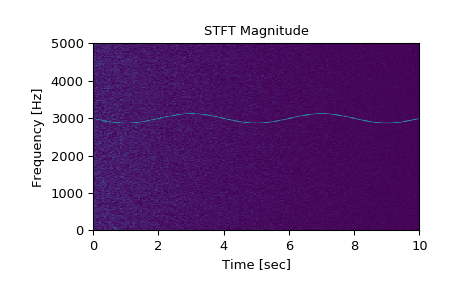# scipy.signal.stft¶

scipy.signal.stft(x, fs=1.0, window='hann', nperseg=256, noverlap=None, nfft=None, detrend=False, return_onesided=True, boundary='zeros', padded=True, axis=-1)[source]

Compute the Short Time Fourier Transform (STFT).

STFTs can be used as a way of quantifying the change of a nonstationary signal’s frequency and phase content over time.

Parameters
xarray_like

Time series of measurement values

fsfloat, optional

Sampling frequency of the x time series. Defaults to 1.0.

windowstr or tuple or array_like, optional

Desired window to use. If window is a string or tuple, it is passed to get_window to generate the window values, which are DFT-even by default. See get_window for a list of windows and required parameters. If window is array_like it will be used directly as the window and its length must be nperseg. Defaults to a Hann window.

npersegint, optional

Length of each segment. Defaults to 256.

noverlapint, optional

Number of points to overlap between segments. If None, noverlap = nperseg // 2. Defaults to None. When specified, the COLA constraint must be met (see Notes below).

nfftint, optional

Length of the FFT used, if a zero padded FFT is desired. If None, the FFT length is nperseg. Defaults to None.

detrendstr or function or False, optional

Specifies how to detrend each segment. If detrend is a string, it is passed as the type argument to the detrend function. If it is a function, it takes a segment and returns a detrended segment. If detrend is False, no detrending is done. Defaults to False.

return_onesidedbool, optional

If True, return a one-sided spectrum for real data. If False return a two-sided spectrum. Defaults to True, but for complex data, a two-sided spectrum is always returned.

boundarystr or None, optional

Specifies whether the input signal is extended at both ends, and how to generate the new values, in order to center the first windowed segment on the first input point. This has the benefit of enabling reconstruction of the first input point when the employed window function starts at zero. Valid options are ['even', 'odd', 'constant', 'zeros', None]. Defaults to ‘zeros’, for zero padding extension. I.e. [1, 2, 3, 4] is extended to [0, 1, 2, 3, 4, 0] for nperseg=3.

Specifies whether the input signal is zero-padded at the end to make the signal fit exactly into an integer number of window segments, so that all of the signal is included in the output. Defaults to True. Padding occurs after boundary extension, if boundary is not None, and padded is True, as is the default.

axisint, optional

Axis along which the STFT is computed; the default is over the last axis (i.e. axis=-1).

Returns
fndarray

Array of sample frequencies.

tndarray

Array of segment times.

Zxxndarray

STFT of x. By default, the last axis of Zxx corresponds to the segment times.

istft

Inverse Short Time Fourier Transform

check_COLA

Check whether the Constant OverLap Add (COLA) constraint is met

check_NOLA

Check whether the Nonzero Overlap Add (NOLA) constraint is met

welch

Power spectral density by Welch’s method.

spectrogram

Spectrogram by Welch’s method.

csd

Cross spectral density by Welch’s method.

lombscargle

Lomb-Scargle periodogram for unevenly sampled data

Notes

In order to enable inversion of an STFT via the inverse STFT in istft, the signal windowing must obey the constraint of “Nonzero OverLap Add” (NOLA), and the input signal must have complete windowing coverage (i.e. (x.shape[axis] - nperseg) % (nperseg-noverlap) == 0). The padded argument may be used to accomplish this.

Given a time-domain signal $$x[n]$$, a window $$w[n]$$, and a hop size $$H$$ = nperseg - noverlap, the windowed frame at time index $$t$$ is given by

$x_{t}[n]=x[n]w[n-tH]$

The overlap-add (OLA) reconstruction equation is given by

$x[n]=\frac{\sum_{t}x_{t}[n]w[n-tH]}{\sum_{t}w^{2}[n-tH]}$

The NOLA constraint ensures that every normalization term that appears in the denomimator of the OLA reconstruction equation is nonzero. Whether a choice of window, nperseg, and noverlap satisfy this constraint can be tested with check_NOLA.

New in version 0.19.0.

References

1

Oppenheim, Alan V., Ronald W. Schafer, John R. Buck “Discrete-Time Signal Processing”, Prentice Hall, 1999.

2

Daniel W. Griffin, Jae S. Lim “Signal Estimation from Modified Short-Time Fourier Transform”, IEEE 1984, 10.1109/TASSP.1984.1164317

Examples

>>> from scipy import signal
>>> import matplotlib.pyplot as plt


Generate a test signal, a 2 Vrms sine wave whose frequency is slowly modulated around 3kHz, corrupted by white noise of exponentially decreasing magnitude sampled at 10 kHz.

>>> fs = 10e3
>>> N = 1e5
>>> amp = 2 * np.sqrt(2)
>>> noise_power = 0.01 * fs / 2
>>> time = np.arange(N) / float(fs)
>>> mod = 500*np.cos(2*np.pi*0.25*time)
>>> carrier = amp * np.sin(2*np.pi*3e3*time + mod)
>>> noise = np.random.normal(scale=np.sqrt(noise_power),
...                          size=time.shape)
>>> noise *= np.exp(-time/5)
>>> x = carrier + noise


Compute and plot the STFT’s magnitude.

>>> f, t, Zxx = signal.stft(x, fs, nperseg=1000)
>>> plt.pcolormesh(t, f, np.abs(Zxx), vmin=0, vmax=amp)
>>> plt.title('STFT Magnitude')
>>> plt.ylabel('Frequency [Hz]')
>>> plt.xlabel('Time [sec]')
>>> plt.show()#### Previous topic

scipy.signal.vectorstrength

#### Next topic

scipy.signal.istft